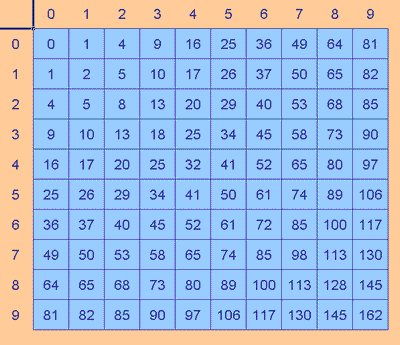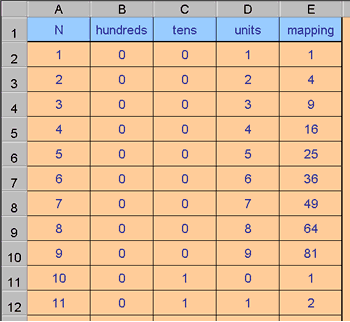# Excel Investigation: Happy Numbers

##### Age 11 to 16 Challenge Level:

Happy Numbers were introduced in this problem from June 1997.

I was first shown Happy Numbers twenty years ago. It works like this:

Chains of numbers are made. The first number in the chain is split into individual digits, each digit is squared and the sum of these squares becomes the next number in the sequence, and so the process continues; splitting, squaring and adding.

For example, starting with 35 the next number is 34, that's 9 + 25, the number after 34 is 25, followed by 29, 85 and so on.

Explore this process for yourself to see what happens.
Do numbers just get larger and larger, or is there a limit?
Do numbers eventually map back to themselves?

Using a spreadsheet for mapping sequences of this kind

Sometimes a mathematical situation seems to have no particular purpose but, if our curiosity is excited, and our minds become energised, then exploring a situation's possibilities may reveal an interesting structure or lead us to invent a technique that could be useful elsewhere. That is what happened for me with this investigation. I should admit that the Happy Numbers investigation itself has never done much to arouse my curiosity. When I found answers, my response was "OK, now I know. So what?" But the investigation did lead me to try out an Excel function which looked up results I had already calculated, and that was a worthwhile discovery.

Putting Excel to work

As always, the main value of the spreadsheet when investigating a mathematical situation, is to give results quickly and easily, so that as much of my attention as possible is available to think about any patterns or connections I notice.

When I first explored Happy Numbers I didn't use a spreadsheet. Actually twenty years ago spreadsheets were strange and new to most people. In some ways that was a good thing. Using a computer can frequently become the automatic choice. When we realise we have become automatic in our response to a situation it can often be helpful to pause and try out the options; at least in our imagination.

Twenty years ago, in my pre-spreadsheet days, I sat for a bit, thought about the process I was being invited to explore, tried out a few calculations, started recording some results, and so on. I still usually start work this way.

After a little while I realised that I was spending too much time calculating and not enough time thinking about what was happening in the process. I did notice that the order of the digits was unimportant, that starting at 35 or 53 was really the same thing apart from the first number. I also invested ten minutes, away from the main task, to made a table of results so that I could at least stop doing all of the individual calculations and record my results faster.

I produced the table back then by doing my own calculations, except for the symmetric values of course. Now I would very likely use a spreadsheet for a table of results from a function of two independent variables.
Click here to see how to do this.

The table of results also helped in another way. It helped me see that all two-digit numbers mapped onto one- or two-digit numbers, except those that mapped onto 100, 106, 113, 128, 130, 145, or 162, but each of these immediately map back to a two-digit result, so the set has closure.

It is also worth pausing to see what happens to numbers with plenty of digits (they always reduce in size), and to understand why that happens.The three-digit number which produces the largest next value is 999, and this maps to 243, so all three-digit numbers map to three-digit numbers until they fall into the two-digit set discussed above.

The four-digit number which produces the largest next value is 9999 which maps to 324, so all four-digit numbers map to numbers with less than four digits.

I said earlier that the Happy Numbers problem did make me think about how to use a spreadsheet in a new way: to look up results I had already calculated.

Here's how I did that:

Happy Numbers with VLOOKUP

Although the square table was useful when I had to fill in the answers myself, because it saved me doing separate calculations for both numbers in a pairs like 35 and 53, once a spreadsheet is doing the calculations this saving is not of much value.
So I produced a table that had the numbers from 1 to 200 in the first column, split these numbers into individual digits, which were then squared and added up.Here's the Excel file for the Happy Numbers mapping .

Isolating Digits

In the Excel file "Happy Numbers mapping" column A contains the value to be mapped, columns B, C, and D contain the individual digits, and column E contains the mapping.

The following explains how the digits are isolated.

You need to understand that the INT function takes the integer part of a value and ignores the rest. INT of 3.845 is just 3.

B2 calculates the number of hundreds using the formula: =INT(A2/100)
A2 was the original value, dividing it by 100 and taking just the integer part of this result reports the number of hundreds in the original number.

For example: 374 divided by 100 is 3.74 whose integer part is 3, the hundreds digit.
C2 calculates the number of tens using the formula: =INT(A2/10)-10*INT(A2/100)
INT(A2/10) calculates the number of tens. For the example of 374 this would be 37.
INT(A2/100) calculates the number of hundreds, 3, as already explained.
So 37 minus 10 lots of 3 gives the result 7, the tens digit as required.
D2 contains the formula: =A2-10*INT(A2/10)
INT(A2/10) is the number of tens, 37 in the example.
So the original number minus 10 lots of the number of tens, 374 - 370, gives 4, the required units digit.

Once I had these basic results available, Excel could look these up as each sequence was created using starting numbers 1 to 200 .

Click here to see the Excel file Happy Numbers .

Click here to see the same Excel file with conditional formatting used to highlight the structure .

Using Conditional Formatting makes values of interest easier to see.

Click here to look at notes on conditional formatting.

When to use the LOOKUP technique

What made this problem different from some other sequence work was that the mapping rule was not a single, simple formula to enter into a spreadsheet cell.

Consider a very different example:

I can find one approximate solution to the equation $x^2 - 7x + 9 = 0$, capable of unlimited refinement, by using the sequence mapping rule:
7 - ( 9 divided by the previous value)

It's easy to tell the spreadsheet to take a number, divide 9 by that number, and subtract that result from 7.

Happy Numbers was harder because the digits needed to be separated first.

Although this could have been part of the Excel formula, it would have been a lot to bundle into a single cell, and the risk of error increases.

Done using the Lookup command instead, the process is easy to understand, the results referred to are laid out in an easy-to-follow fashion and the whole process remains transparent.

For this method it was also necessary that the results formed a closed set. Each result leads to another result from that same set.

This kind of structure helps to keep a process clear, making interesting relationships easier to spot, and explain.